Convert Hexadecimal to Decimal in Bash

Four types of number systems are popular in computer systems. These are Decimal, Binary, Octal and Hexadecimal. The binary system is 2 based and all arithmetic calculations are done by computer in Binary system. It uses only two digits, 0 and 1 for calculation. The number system that we use for general calculation is decimal system which is 10 based. 0 to 9 numbers are used in the decimal system for calculation. The octal number system is 8 based and represented by 0 to 7 digits. The hexadecimal number system is 16 based and it uses 0 to 9 and A to F characters to represents the number. You can easily convert one number to another number system using the bash script. How you can convert Hexadecimal (hex) number to Decimal number in Bash is shown in this tutorial using various examples.

Example-1: Using obase, ibase and bc

One of the simple ways to convert any number system to another number system is to use ibase, obase and bc. Create a bash file named hextodec1.sh and add the following code. According to this example, a hex number will be taken as input and converted into the decimal number based on the value of obase and ibase. Here, obase is set to 10 for converting decimal number, ibase is set to 16 to take the input number as hex number and `bc` command is used for conversion.

#!/bin/bash
echo "Type a hex number"
echo -n "The decimal value of \$hexNum="
echo "obase=10; ibase=16; \$hexNum" | bc

Output:

Run the script with bash command and give any hexadecimal number as input to find out the decimal value.

\$ bash hextodec1.sh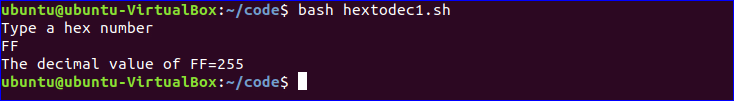Example-2: Using ibase, command line argument and bc

Create a bash file named hextodec2.sh and add the following code. In this example, the input value has to give in the command line argument, which will be read by \$@.  Here, just ibase with 16 value is used to convert hex to the decimal number.

#!/bin/bash
echo -n "The decimal value of \$@="
echo "ibase=16; \$@"|bc

Output:

Run the script with bash command, file name and a hexadecimal number as command line argument. Here, FF is given as command line argument which is taken as hex value.

\$ bash hextodec2.sh FF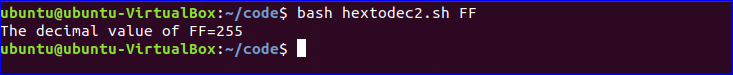Example-3: using printf method

Another option for converting hex to the decimal number is printf. ‘%d’ format specifier is used in printf method to convert any number to decimal number. Create a bash file named hextodec3.sh and add the following code. According to this script, a hex number will be taken as input and it is used in printf method with %d to print the decimal value.

#!/bin/bash
echo "Type a hex number"
printf "The decimal value of \$hexNum=%d\n" \$((16#\$hexNum))

Output:

Run the script with bash command and give any hexadecimal number as input to find out the decimal value.

\$ bash hextodec3.sh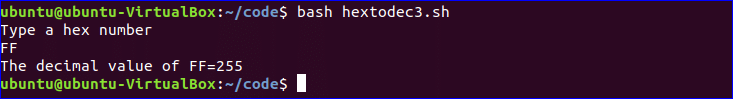Example-4: using double brackets

There is another way to convert hex to the decimal number without using ibase, obase and bc or printf method. You can use double brackets expression with 16 base to convert hex to the decimal number. Create a bash file named hextodec4.sh and add the following code. Here, echo command will take the number as hex and print the output in the decimal number system.

#!/bin/bash
echo "Type a hex number"
echo \$(( 16#\$hexNum ))

Output:

Run the script with bash command and give any hexadecimal number as input to find out the decimal value.

\$ bash hextodec4.sh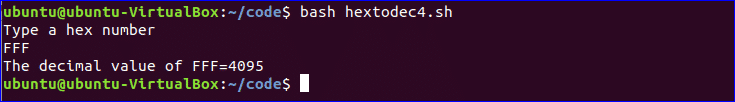Example-5: Converting the list of hexadecimal numbers

Suppose, you have a text file named ‘hexList.txt’ that contains the following list of hex numbers.

HexList.txt
AB05
FF
ABCD
ACCD
BED

Create a bash file named hextodec5.sh and add the following code to convert each hex value of hexList.txt into the decimal value. Here, obase, ibase, and bc are used for conversion. while loop is used to read each hex value from the text file, convert to decimal value and print.

#!/bin/bash
do
echo -n "The decimal value of \$number(Hex)="
echo "obase=10; ibase=16; \$number" | bc
done < hexList.txt

Output:

Run the script with bash command. There are five hex values in the text file and the output shows five decimal values after conversion.

\$ bash hextodec5.sh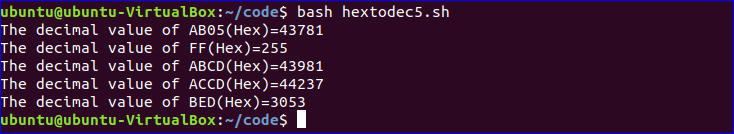This tutorial shows multiple ways to convert hex to decimal values using the bash script. You can follow any of the ways for your conversion purpose. You can also convert other number systems using the scripts mentioned in this tutorial just by changing the base value.